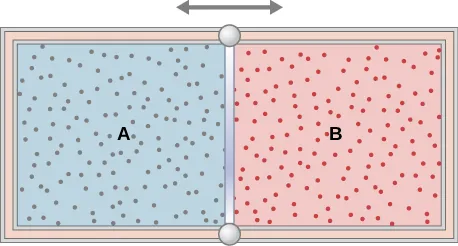University Physics Volume 2

# Challenge Problems

University Physics Volume 2Challenge Problems

### Challenge Problems

94.

One mole of an ideal monatomic gas occupies a volume of $1.0×10−2m31.0×10−2m3$ at a pressure of $2.0×105N/m2.2.0×105N/m2.$ (a) What is the temperature of the gas? (b) The gas undergoes a quasi-static adiabatic compression until its volume is decreased to $5.0×10−3m3.5.0×10−3m3.$ What is the new gas temperature? (c) How much work is done on the gas during the compression? (d) What is the change in the internal energy of the gas?

95.

One mole of an ideal gas is initially in a chamber of volume $1.0×10−2m31.0×10−2m3$ and at a temperature of $27°C27°C$. (a) How much heat is absorbed by the gas when it slowly expands isothermally to twice its initial volume? (b) Suppose the gas is slowly transformed to the same final state by first decreasing the pressure at constant volume and then expanding it isobarically. What is the heat transferred for this case? (c) Calculate the heat transferred when the gas is transformed quasi-statically to the same final state by expanding it isobarically, then decreasing its pressure at constant volume.

96.

A bullet of mass 10 g is traveling horizontally at 200 m/s when it strikes and embeds in a pendulum bob of mass 2.0 kg. (a) How much mechanical energy is dissipated in the collision? (b) Assuming that $CvCv$ for the bob plus bullet is 3R, calculate the temperature increase of the system due to the collision. Take the molecular mass of the system to be 200 g/mol.

97.

The insulated cylinder shown below is closed at both ends and contains an insulating piston that is free to move on frictionless bearings. The piston divides the chamber into two compartments containing gases A and B. Originally, each compartment has a volume of $5.0×10−2m35.0×10−2m3$ and contains a monatomic ideal gas at a temperature of $0°C0°C$ and a pressure of 1.0 atm. (a) How many moles of gas are in each compartment? (b) Heat Q is slowly added to A so that it expands and B is compressed until the pressure of both gases is 3.0 atm. Use the fact that the compression of B is adiabatic to determine the final volume of both gases. (c) What are their final temperatures? (d) What is the value of Q?98.

In a diesel engine, the fuel is ignited without a spark plug. Instead, air in a cylinder is compressed adiabatically to a temperature above the ignition temperature of the fuel; at the point of maximum compression, the fuel is injected into the cylinder. Suppose that air at $20°C20°C$ is taken into the cylinder at a volume $V1V1$ and then compressed adiabatically and quasi-statically to a temperature of $600°C600°C$ and a volume $V2.V2.$ If $γ=1.4,γ=1.4,$ what is the ratio $V1/V2?V1/V2?$ (Note: In an operating diesel engine, the compression is not quasi-static.)

Order a print copy

As an Amazon Associate we earn from qualifying purchases.